# AP Calculus BC Practice Test 27

### Test Information9 questions18 minutes

Calculator Disallowed

1.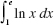equals

2.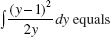3.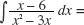4. Given f as graphed, which could be a graph of f ?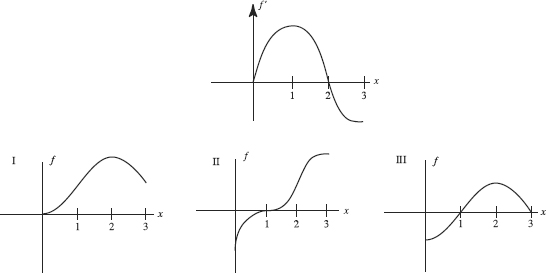5. The first woman officially timed in a marathon was Violet Piercey of Great Britainin 1926. Her record of 3:40:22 stood until 1963, mostly because of a lack of womencompetitors. Soon after, times began dropping rapidly, but lately they have been decliningat a much slower rate. Let M(t) be the curve that best represents winning marathon times in year t. Which of the following is negative?

I. M(t)

II. M (t)

III. M (t)

6.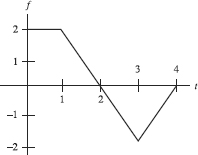The graph of f is shown above. Let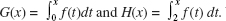Which of the following is true?

7. The minimum value of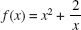on the interval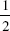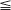x2 is

8.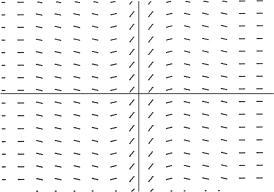Which function could be a particular solution of the differential equation whoseslope field is shown above?

9. A particular solution of the differential equation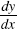= x + y passes through the point (2,1). Using Euler's method with Δx = 0.1, estimate its y-value at x = 2.2.